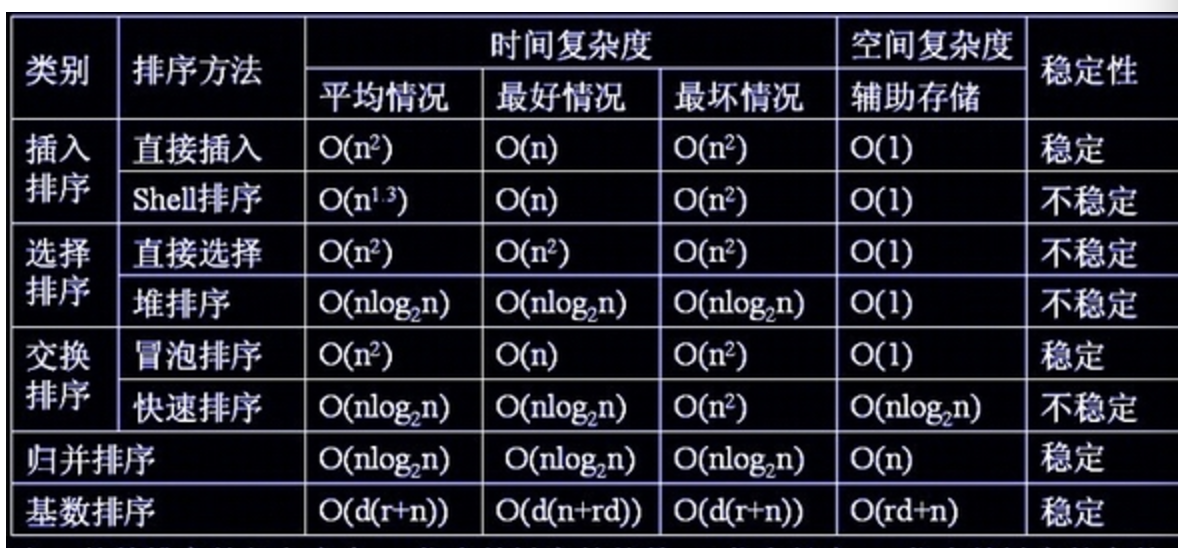# 数据结构 第八章学习小结void ShellPass(SeqList R，int d)
{//希尔排序中的一趟排序，d为当前增量
for(i=d+1;i<=n；i++) //将R[d+1．．n]分别插入各组当前的有序区
if(R[ i ].key<R[i-d].key){
R=R[i];j=i-d； //R只是暂存单元，不是哨兵
do {//查找R的插入位置
R[j+d]=R[j]； //后移记录
j=j-d； //查找前一记录
}while(j>0&&R.key<R[j].key)；
R[j+d]=R； //插入R到正确的位置上
}


posted @ 2020-07-12 23:58  20191003037魏云龙  阅读(168)  评论(0编辑  收藏  举报